# 169 and Level 2

 169 is a composite number and a perfect square. Factor pairs: 169 = 1 x 169 or 13 x 13 Factors of 169: 1, 13, 169 Prime factorization: 169 = 13 x 13 which can be written 13²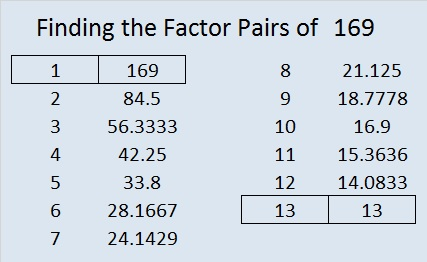—————————————————————–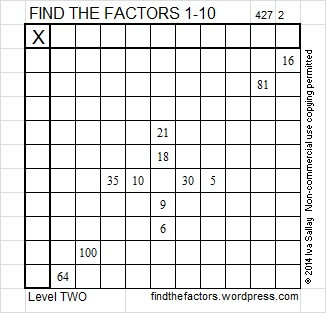Excel file of puzzles and previous week’s factor solutions: 10 Factors 2014-07-07# 49 and V is for Victory Puzzle

49 is a composite number, and it is 7 squared. 49 = 1 x 49 or 7 x 7. Factors of 49: 1, 7, 49. Prime factorization: 49 = 7 x 7 which can also be written 49 = 7².

Since √49 = 7, a whole number, 49 is a perfect square.Whenever 49 is a clue in the FIND THE FACTOR puzzles, write 7 in both the corresponding factor column and factor row.

Level 3 is the first level of the higher level FIND THE FACTORS puzzles. For many people going from level 2 to level 4 would be too overwhelming. Level 3 is a bridge between those two levels and allows a person to move on from the lower levels and victoriously solve a little more difficult puzzle. V is for that victory.

Level 3 puzzles are designed to be solved starting from a row or column at the top of the puzzle with 2 clues. First find the common factor of those two clues that will allow you to write only numbers from 1 to 10 in the first column of the puzzle. Then factor row by row to the bottom of the puzzle making sure each number from 1 to 12 is written only once in both the factor row and the factor column. You will notice a rhythm for the answers as you work.This week’s puzzles are available in an excel file here. If you have a spreadsheet program on your computer, you can access it. If you enable editing in excel, you can type your answers directly onto the puzzle, and you can also easily print the puzzles. May you be victorious in your efforts!

Here are the factors to last week’s level 3 puzzle: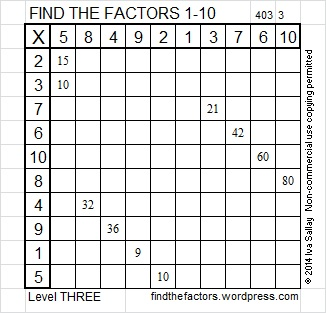This puzzle was solved starting with the column with 2 clues near the top of the puzzle and then factoring row by row until we reached the bottom of the puzzle.

# 36 and The Six Word Challenge

36 is a composite number, and it is 6 squared. 36 = 1 x 36, 2 x 18, 3 x 12, 4 x 9, or 6 x 6. Factors of 36: 1, 2, 3, 4, 6, 9, 12, 18, 36. Prime factorization: 36 = 2 x 2 x 3 x 3, which can also be written 36 = 2² x 3².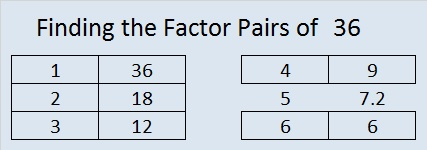Since √36 = 6, a whole number, 36 is a perfect square.

When 36 is a clue in the FIND THE FACTORS  1 – 10 puzzles, use either 4 x 9 or 6 x 6. When 36 is a clue in the FIND THE FACTORS 1 – 12 puzzles, use either 3 x 12, 4 x 9 or 6 x 6. Only one of those combinations will work for any particular puzzle.

I enjoy this multiplication rhyme: Six times six, magic tricks, abracadabra, thirty-six!

A fellow blogger issued a challenge for her readers to write a puzzling story or tell a story about a puzzle using exactly six words. I’m choosing to give puzzle directions today using these six words:

Without guessing, logically decide puzzle’s factors.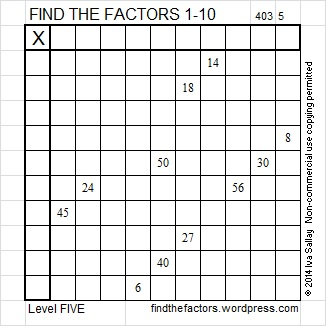It was a fun challenge. Her blog has a 6-word story written by Ernest Hemingway as well as a few written by other bloggers.

This week’s puzzles are also available in an excel file here. If you have a spreadsheet program on your computer, you can access it. If you enable editing in excel, you can type your answers directly onto the puzzle, and you can also easily print the puzzles.

Here are the factors for last week’s level 5 snowflake puzzle:There was more than one way to logically decide the snowflake puzzle’s factors. Some sets of clues needed to be avoided in the beginning: (18, 6) because both can be factored by 2, 3, and 6; (60, 40) because each can be factored by 5 and 10; and (16, 8) because both can be factored by 2, 4, and 8.

The column with 5 clues was a good place to start the puzzle after we narrowed down the common factor that would work for all the clues. The table below shows a way to find all the factors from 1 to 12 using logic without guessing and checking:Related article:

http://drieskewrites.wordpress.com/2014/01/22/six-word-story-challenge-puzzle/

# 25 and Level 3

25 is a composite number, and it is 5 squared. 25 = 1 x 25 or 5 x 5. Factors of 25: 1, 5, 25. Prime factorization: 25 = 5 x 5, which can also be written 25 = 5².

Since √25 = 5, a whole number, 25 is a perfect square.When 25 is a clue in the FIND THE FACTORS puzzles, write 5 in both the corresponding factor row and the factor column.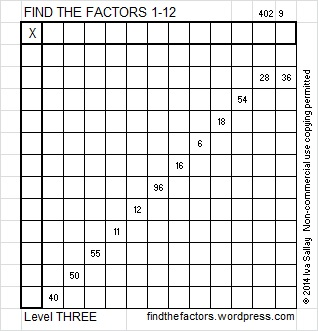Write the numbers 1-12 in the top row and again in the first column so that those numbers are the factors of the given clues. Use logic, not guessing and checking, to find its unique solution. Level 3 puzzles are designed to be solved starting from a row at the top of the puzzle with 2 clues. First find the common factor of those two clues that will allow you to write only numbers from 1 to 12 in the factor row at the top of the puzzle. Then factor row by row to the bottom of the puzzle making sure each number from 1 to 12 is written only once in both the factor row and the factor column. You will notice a rhythm for the answers as you work. Good luck!

This week’s puzzles are also available in an excel file here if you have a spreadsheet program on your computer. If you enable editing in excel, you can type your answers directly onto the puzzle, and you can also easily print the puzzles.

Here is the solution to last week’s level 3 puzzle:# 16 Silver Bells

16 is a composite number, and it is 4 squared. 16 = 1 x 16, 2 x 8, or 4 x 4. Factors of 16: 1, 2, 4, 8, 16. Prime factorization: 16 = 2 x 2 x 2 x 2, which can also be written 16 = 2⁴.Since √16 = 4, a whole number, 16 is a perfect square.

When 16 is a clue in the FIND THE FACTORS puzzles, use either 2 x 8 or 4 x 4. Only one of those sets of factors will work for any particular puzzle.

“Silver bells, silver bells.
It’s Christmas time in the city.”

Find the Factors is a type of logic puzzle. To solve one of the above puzzles, place the numbers 1 – 10 in both the top row and in the first column so that those numbers are factors of the given clues. For each puzzle, there is only one solution. Click on 10 Factors 2013-12-16 to find these and a few more puzzles, as well as last Monday’s solutions.

Some of these Related articles have the lyrics or soundtrack to Silver Bells:

# 4 Rhyme and Rhythm

4  is the first composite number, and it is 2 squared. 4 = 1 x 4 or 2 x 2. Factors of 4: 1, 2, 4. Prime factorization: 4 = 2 x 2, which can also be written 4 = 2².

Since √4 = 2, a whole number, 4 is a perfect square.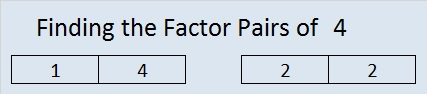When 4 is a clue in the FIND THE FACTORS puzzles, the factors might be 1 and 4 or they might be 2 and 2.

—————————————————————–

Seventeen years ago, when my daughter was learning the multiplication facts, I came across a rhyme that taught one fact:

5, 6, 7, 8……….56 is 7 times 8.

Coincidentally,

1, 2, 3, 4……….I know twelve is 3 times 4.

3 x 4 = 12 isn’t as difficult to remember as 7 x 8 = 56. Still I find it fun to notice the relationship between counting to eight and these two multiplication facts. I enjoyed the rhymes mentioned in my 10/31/2013 post, and I have found yet another site with rhymes for learning the multiplication facts. Two rhymes similar to these 12 and 56 counting rhymes were even included!  The site is : http://www.teacherweb.com/NY/Quogue/MrsLevy/MULTIPLICATION-RHYMES.pdf

Some children have no problem memorizing number facts, but for some children, a rhyme makes learning the facts more fun and much easier.  Even though I already know all of the basic multiplication facts, I am going to memorize these rhymes simply because I personally enjoy them.  I also know I will have at least one opportunity every week to share them with someone trying to memorize the facts: Already when a student asks me, “what’s 7 x 8?” I always answer in rhyme.

Besides the two rhymes listed above, my favorites are:

 Times One: Mirror, mirror look and see, it’s the other number, not me. Times Zero:  Zero is always the hero Six times six / Magic tricks / Abracadabra / thirty-six A tree on skates fell on the floor / Three times eight is twenty-four. A 4 by 4 is a big machine, Iʼm going to get one when Iʼm 16.

This week I even wrote one myself:  Twelve times twelve / Is a dozen dozen / A gross one forty-four / Just ask my cousin.

When a very young child loves a rhyme, he or she will want to hear it over and over again. Mother Goose rhymes have been enriching lives for years. Being able to fully comprehend what the rhyme is about isn’t necessary at first so even preschoolers can be introduced to these rhymes.

Robin Liner writes a blog (crazygoodreaders.wordpress.com) that discusses reading and dyslexia.  On October 5, 2013 , she wrote Rhythm and Rhyme: A Phonological Power Tool.  She wrote, “Rhymes provide subconscious clues.” That means someone is more likely to get an answer right when that answer rhymes with the question. What a fun and powerful way to learn!

Much of what she wrote not only applies to learning to read but also to learning math, science, history, ……. anything.

Twice a week I put 6 new puzzles in an excel file that is attached to this blog. The puzzles can be solved using logic and knowledge of the multiplication table. Here is one of the puzzles I created this week.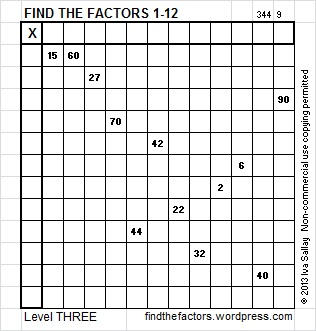Click on this link, 12 Factors 2013-11-07,  for more puzzles and the previous week’s solutions. How do you solve the puzzles? Place the numbers 1 – 12 in both the top row and the first column so that those numbers are the factors of the given clues.

Update: I like the idea of using rhymes to learn multiplication facts so much that I compiled my own list: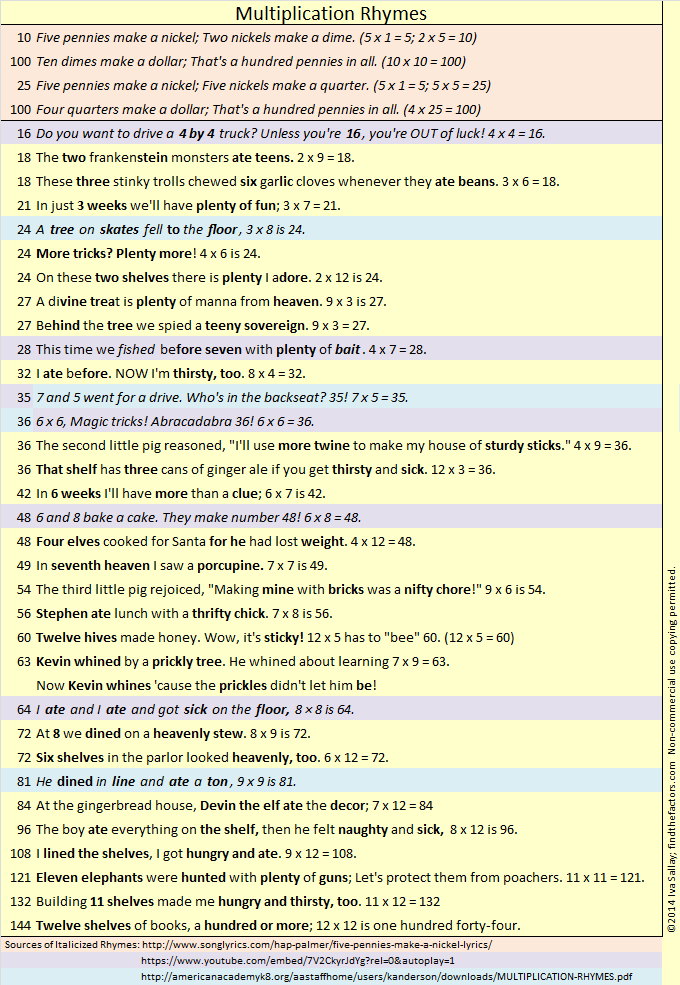# 1 Perfect Square

1 has 1 factor. 2 has 2 factors…that is the end of that pattern because no number greater than 2 equals its number of factors.

• 1 is not a prime number, and 1 is not a composite number. 1 is in a category all by itself. It is classified as a unit.
• 1 has no Prime factorization.
• p⁰ = 1, where p is any prime number, so 1 is a factor of every prime number and every composite number.
• 1 is also the only number to have exactly 1 factor.
• Factors of 1: 1
• Factor pairs: 1 = 1 x 1
• √1 = 1. Since its square root is a whole number, 1 is a perfect square.1(n) = n and n ÷ 1 = n for every number n.

Also 1⁰ = 1, 1¹ = 1, 1² = 1, 1³ = 1, 1⁴ = 1, 1⁵ = 1, 1⁶ = 1, 1⁷ = 1, 1⁸ = 1, 1⁹ = 1. In fact, 1 raised to any power equals 1. Even 1⁻⁹⁸⁷⁶⁵⁴³²¹⁰ = 1.

Not only that, but any number (EXCEPT 0) raised to the zeroth power is equal to 1.

One of my college professors wrote something like the following on the board to show why 0º is NOT defined: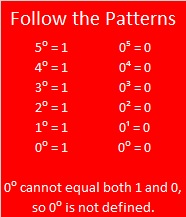When 1 is a clue in the FIND THE FACTORS puzzle, write 1 in both the corresponding factor row and the corresponding factor column.

————————————————————————

Like most people, you probably know how to fill in a multiplication table even if it looks like this:The numbers that are given on a table can be called clues. The table above has 20 clues. What is the least number of clues that a table could have and still only have one way to fill it out?Although the table above has just nine clues, there is still only one way to complete it. Nine is the fewest number of clues that will still yield a unique solution.  All of those clues would have to be perfect squares: 1, 4, 9, 16, 25, 36, 49, 64, 81, and 100. One of the clues will be missing, but it isn’t difficult to figure out where the missing clue should go.  Always find the factors on the outside of the puzzle BEFORE writing down the products on the inside of the puzzle.

The puzzle above is rated difficulty level ONE because you only need to know 10 multiplication facts to find all the factors. If this puzzle is too easy for you, you can try a more difficult puzzle. Levels FOUR, FIVE, or SIX will be much more challenging, even for adults.

This link, 10 Factors 2013-10-28, will bring up an excel file with the puzzles that are on this post.  After you enable editing, you can print the puzzles or type the factor answers directly onto the excel file.

An answer key will be posted one week after a puzzle is published.

If you don’t want to open the excel file, the rest of the puzzles will be printed below. If you cut and paste them on a document, you can make them any size you want.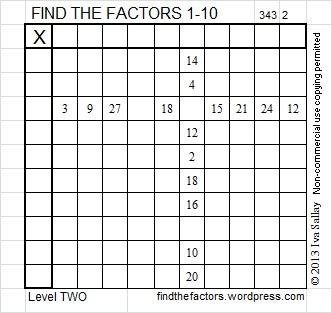If you want to check your work, the answers are given in a tab of the excel file that was published a week later: 10 factors 2013-11-04.